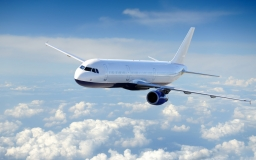Aircraft angines

The two engines of the aircraft are enough to supply the fuel for five hours of operation. However, one of the engines has a malfunction and thus consumes one-third more fuel. How long can the plane be in the air before it runs out of fuel? After an hour of malfunctioning, the engine will resume normal operation. How much time can a total aircraft spend in the air?

t2 =  4.2857 h
t3 =  4.8333 h

Step-by-step explanation:Did you find an error or inaccuracy? Feel free to write us. Thank you!Tips to related online calculators
Looking for calculator of harmonic mean?
Looking for a statistical calculator?
Need help to calculate sum, simplify or multiply fractions? Try our fraction calculator.
Do you have a linear equation or system of equations and looking for its solution? Or do you have a quadratic equation?
Tip: Our volume units converter will help you with the conversion of volume units.